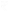# Bank of Maharashtra Emi calculator for Loans

BOM Calculator helps you to calculate monthly EMI with graphs, Total amount with Interest, Total interest amount & yearly interest amount with loan calculator of Bank of Maharashtra.

Calculate Loans EMI Online

Loan Amount
Interest Rate (Reducing) % Per Annum
Loan Tenure (in Months)

Monthly EMITotal Amount with InterestTotal Interest AmountYearly Interest Amount